← Back to all posts

# Neural Network Architectures and Techniques

August 21, 2020

A quick cheat sheet I threw together about things that I wanted to remember as I was taking a course on deep learning.

## Architectures

### Convolutional Networks

Convolutional networks work by applying a filter or set of filters to input data. Convolutions are usually followed by pooling. The net effect of convolution usually reduces the width and height (spatial) dimensions of your data, but increases the number of channels.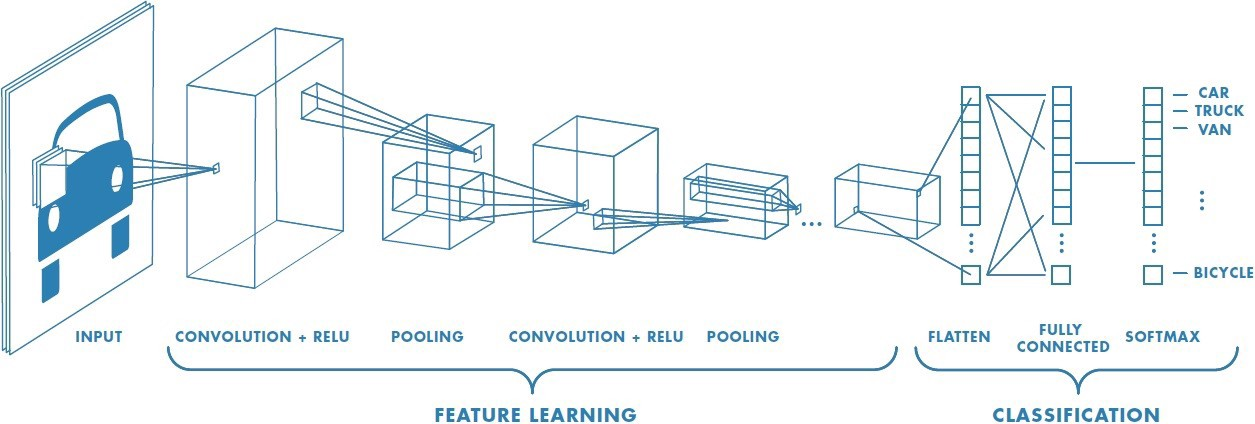Convolutional networks often have a set of convolution + activation + pooling layers, which get trained to learn features. We observe how while the spatial dimensions of each layer decrease, the number of channels increase. After these convolution layers, the last is flattened and fed into a set of fully connected layers. While convolutional layers will often use the relu activation function, the final fully connected layer will use a softmax layer (in the case of classification problems).

#### Filter Size

The size of the filter determines the number of values over which the filter is applied. Usually odd numbered filter sizes are used.

#### Stride

The stride determines how many values are moved over each application of the filter.

Padding adds 0 to the edges of the spatial dimensions of the data.

• Same padding is adding enough padding such that the filter’s center covers even the corner values. This is equal to floor(filter size / 2).

#### Pooling

Convolution is usually followed by pooling. Pooling can also be thought of as another convolution, but where the function is not the element wise product of the filters but as either

• Mean: takes the average of elements within the pooling size.
• Max: takes the maximum value of elements within the pooling area. Used more often.

### Inception Networks

Inception networks compute convolutions and poolings of different sizes, and then append all these values together. This lets the next layer of the network learn to choose which convolution outputs to use, as which are the most meaningful.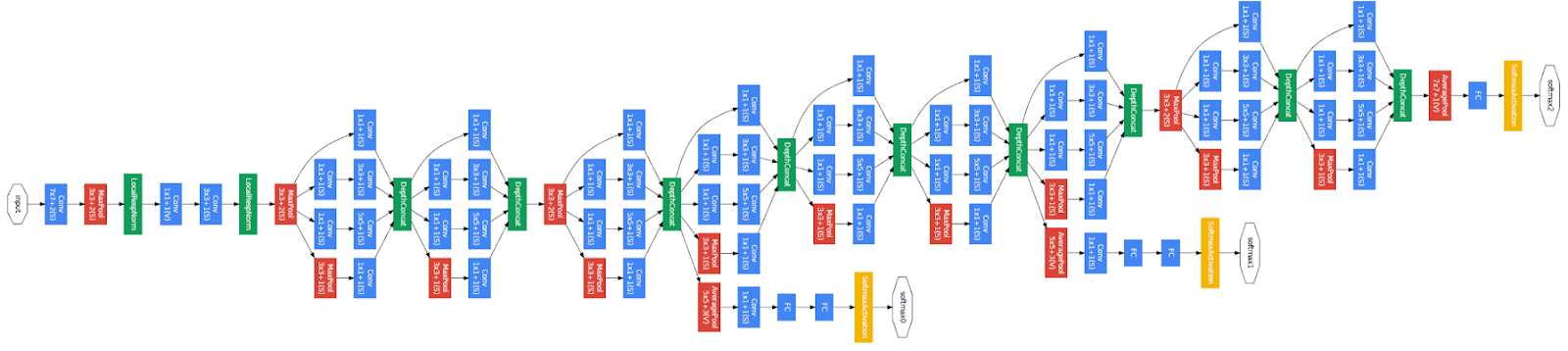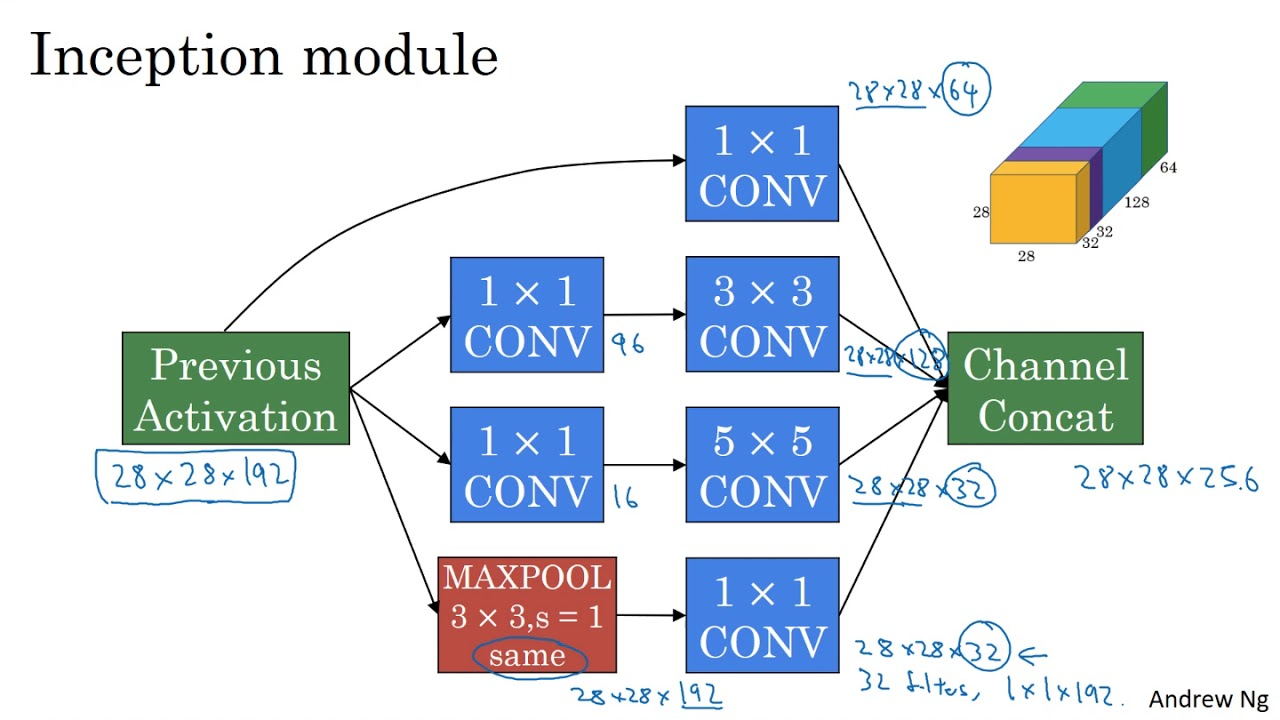### Residual Networks

Residual networks allow information from earlier in the network to pass into later sections of the network. The activation function computed for one layer can “skip” directly into a deeper layer of the network, and be added to that layer’s value before activation is computed. This skip can be one or more layers.

Residual networks allow a neuron to essentially learn the identity function for its input, by setting F(x) = 0 such that it outputs only x from before. This has a similar effect as L2 regularization, where essentially that neuron performs no new operation.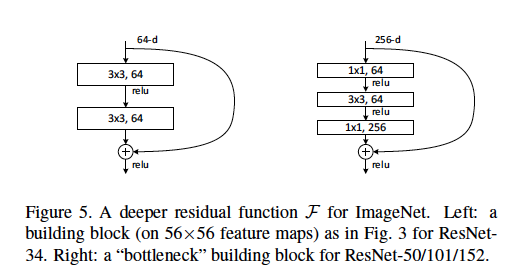#### Identity Layers

Identity layers are as shown in the diagram above. These are used when the dimensions of the weight layers are the same. X is simply passed in and added to F(x).

#### Convolution Layers

Convolutional layers are the same in principle, but use an additional convolution on the x passed through in order to resize it to match the dimension of F(x).

## Techniques

### Regularization

Regularization solves the issue of overfitting to the training set, causing your model not to generalize well.

### L2 Regularization

L2 regularization is the most often used type of regularization, and it works by penalizing weights that become too large. In calculating the cost function, it adds the loss function L to the magnitude of the weights, scaled by a regularization factor lambda. By penalizing larger weights, L2 regularization tries to ensure that the function fitted to the data is as simple as possible. This reduces the risk of overfitting to test data.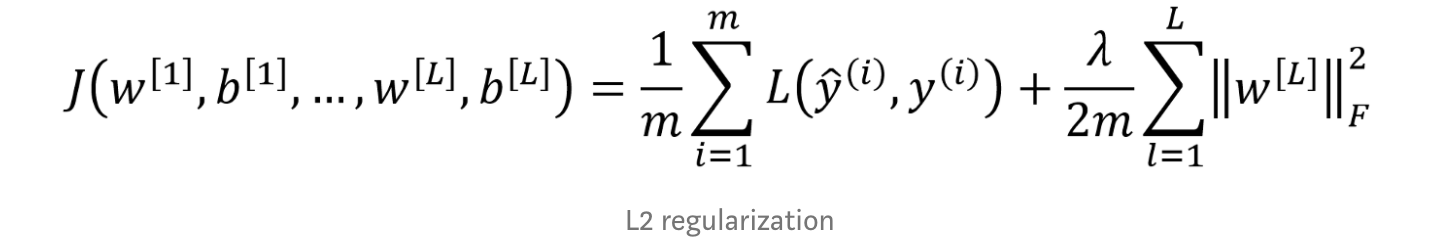### Dropout Regularization

In training, dropout randomly removes neurons from layers. This forces the network to redistribute weighting of parameters, and that the network does not become too reliant on one single neuron. The rate at which neurons are turned off by dropout is governed by the dropout threshold.### Batch Normalization

Batch norm normalizes the mean and variance of each layer’s activation (thereby fixing the mean and variance of the next layer’s inputs). Batch norm is often used in residual networks.